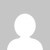# Python capitalize the first letter of every word in the list | Example code

Use Python capitalize() method to capitalize every first letter of a word in the list. You have to also use for loop to iterate through the list.

``str.capitalize()``

## Example How tocapitalize the first letter of every word in the list

Python simple code.

``````list1 = ["Hello", "how", "are", "you"]

for i in range(len(list1)):
list1[i] = list1[i].capitalize()

print(list1)
``````

Output:

### Another example

You can also use the python string title method with the same process.

``````list1 = ["Hello", "how", "are", "you"]

for i in range(len(list1)):
list1[i] = list1[i].title()

print(list1)
``````

Output: [‘Hello’, ‘How’, ‘Are’, ‘You’]

Do comment if you have any doubts and suggestions on this python list topic.

Note: IDE: PyCharm 2021.3.3 (Community Edition)

Windows 10

Python 3.10.1

All Python Examples are in Python 3, so Maybe its different from python 2 or upgraded versions.

## 3 thoughts on “Python capitalize the first letter of every word in the list | Example code”

1.Hi
why do you use [i] in list1[i] = list1[i].capitalize() ? what does it mean?

1.It capitalizes the first index value. list1[i] means get first index value.

2.Hi
why do you use [i] in <> ? what does it mean?

This site uses Akismet to reduce spam. Learn how your comment data is processed.